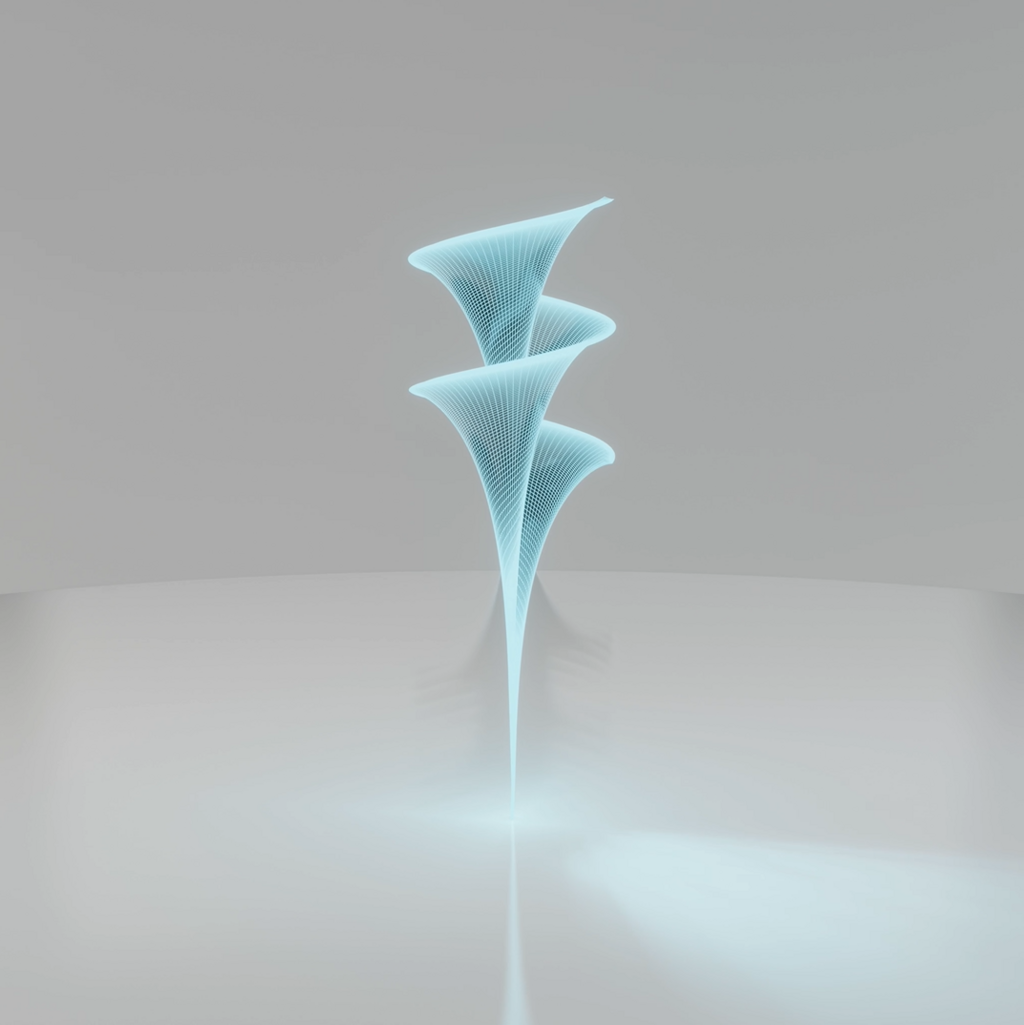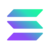This website needs js to run.
Universe\$0.004536

0.19%Presenting to you a visualization of mathematically made objects using XYZ functions. Visualizations are 8 seconds long, 1250px-1250px, 30fps, .mp4, first Drop includes 24 objects. Enjoy investing and flipping. Discord Server coming soon!

208Views

X:

(sinh(v)*cos(3*u))/(1+cosh(u)*cosh(v))

Y:

(cosh(v)*sinh(u))/(1+cosh(u)*cosh(v))

Z:

(sinh(v)*sin(3*u))/(1+cosh(u)*cosh(v))

X: (sinh(v)*cos(3*u))/(1+cosh(u)*cosh(v)) Y: (cosh(v)*sinh(u))/(1+cosh(u)*cosh(v)) Z: (sinh(v)*sin(3*u))/(1+cosh(u)*cosh(v))
cgi
animation
Hyper HelicoidalLicense: Pub/NonCommMintedon SolSea2
Gold
Doublecheck everything before you buy!How to spot fakes?
• ###### Bids
Royalties on secondary sales: 2.5 %
Listed by: 7tKM...xG8d